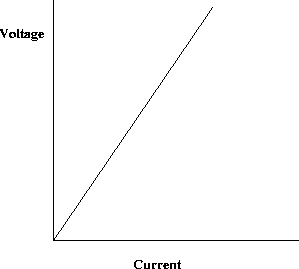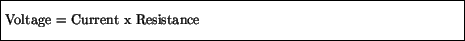Next: Electrical Power Up: Charges in motion Previous: Current

## Resistance

For most materials, there is a simple relationship between the potential difference applied across two points and the current generated. An Ohmic material is one for which the potential difference and current are related as below:For such materials the voltage and current are proportional - doubling the potential difference doubles the current. The constant of proportionality is called the resistance, which is defined through Ohm's law:The units of resistance are Volts / Ampères, or Ohms (). Thus, for a given potential difference, materials with a high resistance will allow a small current relative to a material with a low resistance.

In analogy with heat resistance and conductivity, one can define an electrical conductivity as being proportional to the inverse of the resistance. Thus, good electrical conductors, such as copper, have a low resistance, and poor electrical conductors, such as concrete, have a high resistance.

At the atomic level, currents are pictured as the flow of the outer electrons of atoms through the material. Resistance then results from collisions of electrons with other electrons and with atoms. From this we would expect that raising the temperature of a material would increase the resistance, as the added heat energy would cause the electrons to move faster and hence collide more often. This is indeed what is generally observed.Next: Electrical Power Up: Charges in motion Previous: Current
modtech@theory.uwinnipeg.ca
1999-09-29